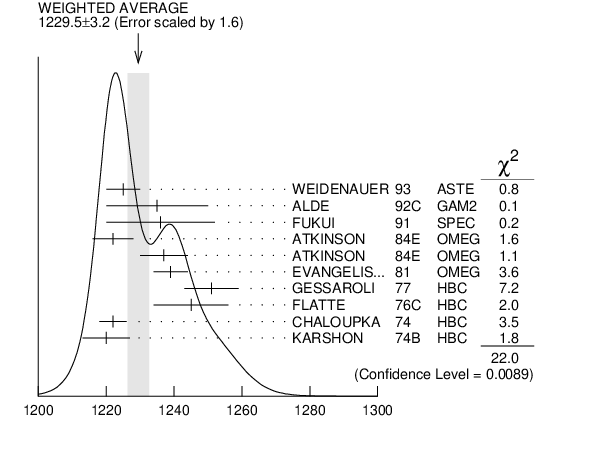#### ${{\boldsymbol b}_{{1}}{(1235)}}$ MASS

VALUE (MeV) EVTS DOCUMENT ID TECN CHG  COMMENT
 $\bf{ 1229.5 \pm3.2}$ OUR AVERAGE  Error includes scale factor of 1.6. See the ideogram below.
$1225$ $\pm5$
 1993
ASTE ${{\overline{\mathit p}}}$ ${{\mathit p}}$ $\rightarrow$ 2 ${{\mathit \pi}^{+}}$2 ${{\mathit \pi}^{-}}{{\mathit \pi}^{0}}$
$1235$ $\pm15$
 1992 C
GAM2 38,100 ${{\mathit \pi}^{-}}$ ${{\mathit p}}$ $\rightarrow$ ${{\mathit \omega}}{{\mathit \pi}^{0}}{{\mathit n}}$
$1236$ $\pm16$
 1991
SPEC 8.95 ${{\mathit \pi}^{-}}$ ${{\mathit p}}$ $\rightarrow$ ${{\mathit \omega}}{{\mathit \pi}^{0}}{{\mathit n}}$
$1222$ $\pm6$
 1984 E
OMEG $\pm{}$ 25$-$55 ${{\mathit \gamma}}$ ${{\mathit p}}$ $\rightarrow$ ${{\mathit \omega}}{{\mathit \pi}}$ X
$1237$ $\pm7$
 1984 E
OMEG 0 25$-$55 ${{\mathit \gamma}}$ ${{\mathit p}}$ $\rightarrow$ ${{\mathit \omega}}{{\mathit \pi}}$ X
$1239$ $\pm5$
 1981
OMEG - 12 ${{\mathit \pi}^{-}}$ ${{\mathit p}}$ $\rightarrow$ ${{\mathit \omega}}{{\mathit \pi}}{{\mathit p}}$
$1251$ $\pm8$ 450
 1977
HBC - 11 ${{\mathit \pi}^{-}}$ ${{\mathit p}}$ $\rightarrow$ ${{\mathit \pi}^{-}}{{\mathit \omega}}{{\mathit p}}$
$1245$ $\pm11$ 890
 1976 C
HBC - 4.2 ${{\mathit K}^{-}}$ ${{\mathit p}}$ $\rightarrow$ ${{\mathit \pi}^{-}}{{\mathit \omega}}{{\mathit \Sigma}^{+}}$
$1222$ $\pm4$ 1400
 1974
HBC - 3.9 ${{\mathit \pi}^{-}}{{\mathit p}}$
$1220$ $\pm7$ 600
 1974 B
HBC + 4.9 ${{\mathit \pi}^{+}}{{\mathit p}}$
• • We do not use the following data for averages, fits, limits, etc. • •
$1190$ $\pm10$
 1989
DM2 $\pm{}$ ${{\mathit e}^{+}}$ ${{\mathit e}^{-}}$ $\rightarrow$ 5 ${{\mathit \pi}}$
$1213$ $\pm5$
 1984 C
OMEG 0 20$-$70 ${{\mathit \gamma}}{{\mathit p}}$
$1271$ $\pm11$
 1984
SPEC + 200 ${{\mathit \pi}^{+}}$ ${}^{}\mathrm {Z}$ $\rightarrow$ ${}^{}\mathrm {Z}$ ${{\mathit \pi}}{{\mathit \omega}}$${{\mathit b}_{{1}}{(1235)}}$ mass (MeV)
References:
 WEIDENAUER 1993
ZPHY C59 387 ${{\mathit N}}{{\overline{\mathit N}}}$ Annihilation at Rest into Five Pions
 ALDE 1992C
ZPHY C54 553 Study of the ${{\mathit \omega}}{{\mathit \pi}^{0}}$ System
 FUKUI 1991
PL B257 241 Study of ${{\mathit \omega}}{{\mathit \pi}^{0}}$ System in the ${{\mathit \pi}^{-}}{{\mathit p}}$ Charge Exchange Reaction at 8.95 ${\mathrm {GeV/}}\mathit c$
 AUGUSTIN 1989
NP B320 1 Study of the ${{\mathit J / \psi}}$ Decay into Five Pions
 ATKINSON 1984C
NP B243 1 A Spin Parity Analysis of the ${{\mathit \omega}}{{\mathit \pi}^{0}}$ Enhancement Photoproduced in the Energy Range 20 to 70 GeV
 ATKINSON 1984E
PL 138B 459 Inclusive Photoproduction of ${{\mathit \delta}{(980)}}$ and ${{\mathit B}{(1235)}}$ at High x$_{F}$
 COLLICK 1984
PRL 53 2374 Primakoff Production of the ${{\mathit b}_{{1}}{(1235)}}$ Meson
 EVANGELISTA 1981
NP B178 197 Results from a ${{\mathit \pi}^{-}}{{\mathit p}}$ Slow Proton Trigger Experiment at 12 ${\mathrm {GeV/}}\mathit c$
 GESSAROLI 1977
NP B126 382 Analysis of the ${{\mathit \omega}}{{\mathit \pi}^{-}}$ System Produced in the Reaction ${{\mathit \pi}^{-}}$ ${{\mathit p}}$ $\rightarrow$ ${{\mathit \pi}^{-}}{{\mathit \pi}^{-}}{{\mathit \pi}^{+}}{{\mathit \pi}^{0}}$ ${{\mathit p}}$ at 11.2 ${\mathrm {GeV/}}\mathit c$
 FLATTE 1976C
PL 64B 225 Observation of the ${{\mathit B}}$ Meson in ${{\mathit K}^{-}}{{\mathit p}}$ Interactions at 4.2 ${\mathrm {GeV/}}\mathit c$
 CHALOUPKA 1974
PL 51B 407 Production and Decay Properties of the ${{\mathit B}}$ Meson
 KARSHON 1974B
PR D10 3608 Production and Decay Mechanism of the ${{\mathit B}}$ Meson and the ${{\mathit \omega}}{{\mathit \pi}}$ System in ${{\mathit \pi}^{+}}{{\mathit p}}$ Interactions at 5 ${\mathrm {GeV/}}\mathit c$